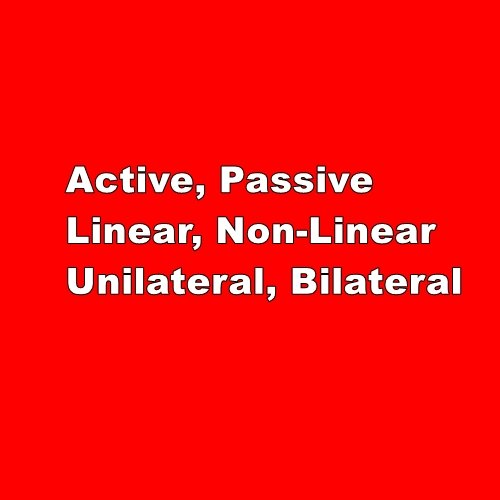# Active,Passive,Linear,Non-Linear,Unilateral, Bilateral Elements

## What are electrical elements

All those elements which provide, consume, transfer or control the electrical energy. Called the electrical elements.

## Classification of electrical elements

There are many types of electrical elements, but some main classifications are –

### 1.On the basis of energy transfer

there are two types of electrical elements on the basis of energy transfer

#### 1. Source

Source– those elements which provide supply in the circuit called source.

Here you are thinking what I am saying about source and load. Anyone know about source and load. but, it is not easy to say which are the source and which are load in any element of the circuit. actually, source and load are determined by the direction of voltage and current. If the direction of voltage and source are the same in any element then that element is called the Source in that circuit.  And if the direction of voltage and current are opposite in any elements then it called the Load.

### 2. On the basis of waveform

#### Active Components or Active Elements

All those components that can change the waveform of electrical energy, called Active Elements.  Active components can process and amplify the electrical signal. Active components can deliver or control the power by the help of other electrical signals. For example, I give a signal of a sinusoidal waveform at diode input,  but the diode gives the output of rectified and I got the non-sinusoidal wave, But if I give a signal at any resistor, any inductor or capacitor then the output from these are the same waveform as input. Diode, Transistor, SCR, IC, and other semiconductor components are Active components.

Graph of the active component is- -ve slope and lie in the 2nd / 4th quadrant.

#### Passive Components or Passive Elements

Passive components can’t process and can’t amplify the electrical signal.  Passive components didn’t deliver or control the power, it consumes the power. Those elements can’t change the waveform of electrical energy, called Passive elements.

Graph of Passive component is lie in 1st /3rd quadrant and +ve slope

Example – Resistor (R), Inductor(L), and Capacitor are Passive components. All possible electrical components except R, L and C are active components.

### 3. On the basis of V-I  Characteristics

#### Linear Elements (We also say Ohmic Element)

A linear circuit is one whose parameters are constant with the time also they do not change with voltage or current & circuit follows Ohm’s Law. V-I characteristics of a linear circuit is a straight line.

Resistors, capacitors, Transformers, and Inductors are Linear components.

#### Non-Linear Elements (It is also called Non-Ohmic element)

A Non-Linear circuit or non-linear element is one whose parameters vary with the time and change with voltage and current and also they don’t follow the Ohm’s Law. V-I characteristics of the non-Linear circuit is Not a straight line.### 4. Unilateral and Bilateral

#### Unilateral Element/Component

If the polarity of the voltage is changed then the characteristics of V-I is also changed then the element is bilateral.  In Unilateral if I change the terminal of that component then the properties of the circuit changed. For example a diode – if we connect diode by changing its terminals then current flowing stopped because diode conducts current in only one direction, so direction and properties of that circuit will be changed, so I can say that diode is a Unilateral element.

All semiconductors devices are unilateral But DIAC is Bilateral .

#### Bilateral Element/Component

Bilateral circuit- If the polarity of the voltage is changed then the characteristics of V-I  remain same, then the element is bilateral. circuit is that circuit whose properties don’t change with the direction.-In the Bilateral element, the voltage-current relation is not the same for both directions. This means if the element changed in other direction then the voltage and current relation don’t change. For example a resistor- if I connect any resistor with changed terminals then I see no effect on the system. The best example of a bilateral element is a transmission line or any conducting wire. if I connect the wire by the changing of its terminals. Then no any change occurs in the circuit.

In the case of the generalized plane, the characteristics of the Bilateral element is always Symmetrical about the origin.

Note – All linear elements are bilateral but the reverse is not true.